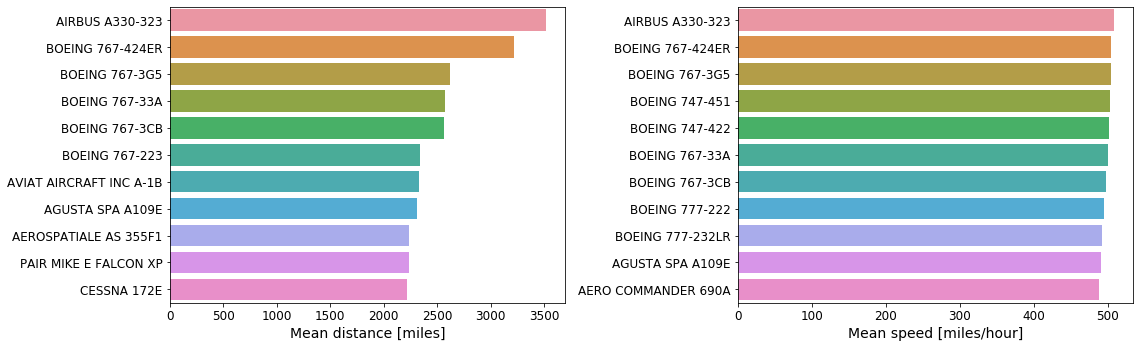# Exploratory Data Analysis: US Airlines 1988 - 2018¶

In :
import vaex
from vaex.ui.colormaps import cm_plusmin

import numpy as np
import pylab as plt
import seaborn as sns


### Adjusting matplotlib parameters¶

In :
SMALL_SIZE = 12
MEDIUM_SIZE = 14
BIGGER_SIZE = 16

plt.rc('font', size=SMALL_SIZE)          # controls default text sizes
plt.rc('axes', titlesize=SMALL_SIZE)     # fontsize of the axes title
plt.rc('axes', labelsize=MEDIUM_SIZE)    # fontsize of the x and y labels
plt.rc('xtick', labelsize=SMALL_SIZE)    # fontsize of the tick labels
plt.rc('ytick', labelsize=SMALL_SIZE)    # fontsize of the tick labels
plt.rc('legend', fontsize=SMALL_SIZE)    # legend fontsize
plt.rc('figure', titlesize=BIGGER_SIZE)  # fontsize of the figure title


### Obtaining the data¶

The original data can be downloaded from United States Department of Transportation. The selected columns are the same as in this older, better known dataset used for competitions available here.

Please refer to this notebook on how to converd the raw data to the memory-mappable HDF5 format.

In :
# read in the data
df = vaex.open('./original_data/hdf5/airline_data_1988_2018.hd5')


### Quick insights into the dataset¶

In :
# Get a high level overview of the DataFrame
df.describe()

Out:
Year Month DayOfMonth DayOfWeek UniqueCarrier TailNum FlightNum Origin Dest CRSDepTime ... Diverted CRSElapsedTime ActualElapsedTime AirTime Distance CarrierDelay WeatherDelay NASDelay SecurityDelay LateAircraftDelay
dtype int16 int8 int16 int8 str str int32 str str int16 ... int8 int32 int32 int32 int32 int32 int32 int32 int32 int32
count 183821926 183821926 183821926 183821926 183821926 147156816 183821926 183821926 183821926 183821925 ... 183821926 183795899 180136665 144694075 183821926 19923595 19923595 19923595 19923595 19923595
NA 0 0 0 0 0 36665110 0 0 0 1 ... 0 26027 3685261 39127851 0 163898331 163898331 163898331 163898331 163898331
mean 2003.6859476273794 6.513381216558464 15.728390529430097 3.9381597057143227 -- -- 1684.4972291118308 -- -- 1332.076048920715 ... 0.0023179171781716615 126.51852634644476 124.53201087074639 105.60806368885527 730.8989127553805 16.530673053733526 2.874630406811622 15.328259533482788 0.09065989345798287 21.535320106637382
std 8.73569 3.42119 8.78432 1.99011 -- -- 1644.95 -- -- 475.781 ... 0.0480889 70.2509 70.2617 68.4631 567.437 44.2425 19.7938 29.8544 2.39507 40.4384
min 1988 1 1 1 -- -- 0 -- -- 0 ... 0 -162 -719 -2378 0 0 0 -60 0 0
max 2018 12 31 7 -- -- 9912 -- -- 2400 ... 1 1865 1440 1399 4983 2580 2692 1848 987 2454

7 rows × 29 columns

### Filtering on the base df.describe()¶

This filters out the unphisical measurements of durations and distances.

In :
# Keep missing values if they fall within the selection criteria.
df_filtered = df[((df.ActualElapsedTime>=0).fillmissing(True)) &
((df.CRSElapsedTime>=0).fillmissing(True)) &
((df.AirTime>0).fillmissing(True)) &
((df.Distance > 0).fillmissing(True))]

# Describe the filtered dataset
df_filtered.describe()

Out:
Year Month DayOfMonth DayOfWeek UniqueCarrier TailNum FlightNum Origin Dest CRSDepTime ... Diverted CRSElapsedTime ActualElapsedTime AirTime Distance CarrierDelay WeatherDelay NASDelay SecurityDelay LateAircraftDelay
dtype int16 int8 int16 int8 str str int32 str str int16 ... int8 int32 int32 int32 int32 int32 int32 int32 int32 int32
count 183819525 183819525 183819525 183819525 183819525 147154521 183819525 183819525 183819525 183819524 ... 183819525 183793498 180134408 144691886 183819525 19923088 19923088 19923088 19923088 19923088
NA 0 0 0 0 0 36665004 0 0 0 1 ... 0 26027 3685117 39127639 0 163896437 163896437 163896437 163896437 163896437
mean 2003.6859480242917 6.513388210528778 15.728389544037828 3.938158647727982 -- -- 1684.4516931539238 -- -- 1332.0765261583422 ... 0.0023172511189983765 126.51923827033315 124.53273411818135 105.61354762491658 730.9031452507561 16.529847431281738 2.8741544483465615 15.32819796810615 0.0906549225702361 21.535796509055224
std 8.73574 3.42119 8.78432 1.99011 -- -- 1644.9 -- -- 475.78 ... 0.048082 70.2508 70.2613 68.4402 567.439 44.2407 19.7918 29.8528 2.39494 40.4387
min 1988 1 1 1 -- -- 0 -- -- 0 ... 0 0 0 1 6 0 0 -60 0 0
max 2018 12 31 7 -- -- 9912 -- -- 2400 ... 1 1865 1440 1399 4983 2580 2692 1848 987 2454

7 rows × 29 columns

## General Exploratory Data Analysis¶

##### Number of flights per year¶

Let's start by looking at the number of scheduled flights per year.

In :
flights_years = df_filtered.Year.value_counts()

plt.figure(figsize=(18,4))
sns.barplot(x=flights_years.index, y=flights_years.values)
plt.xlabel('Year')
plt.ylabel('Total number of flights')
plt.xticks(rotation='vertical')
plt.show()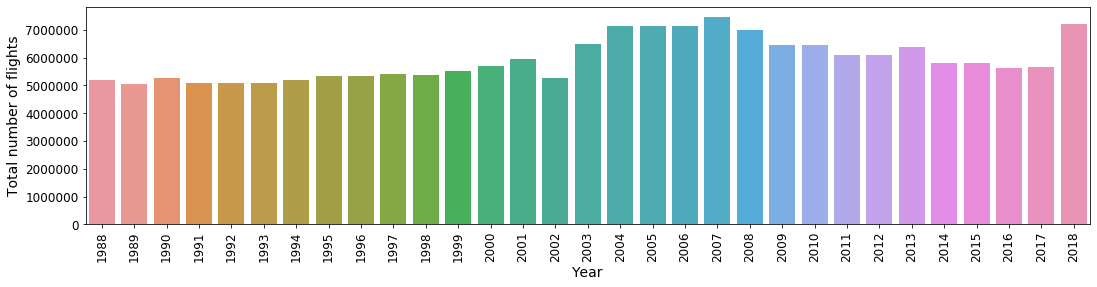The dip in flights in 2002 is due to new regulations following the tragedy in 2001. After that, a year or adjustments to the new protocols, number of flights started increasing again.

##### The most busy airports¶

Find the most busy airports

In :
origin_value_counts = df_filtered.Origin.value_counts()
dest_value_counts = df_filtered.Origin.value_counts()

plt.figure(figsize=(12, 5))

plt.subplot(121)
sns.barplot(x=origin_value_counts.index[:11], y=origin_value_counts.values[:11])
plt.title('Most frequent origins')
plt.xlabel('Airport code', fontsize=14)
plt.ylabel('Number of take-offs')

plt.subplot(122)
sns.barplot(x=dest_value_counts.index[:11], y=dest_value_counts.values[:11])
plt.title('Most frequent destinations')
plt.xlabel('Airport code')
plt.ylabel('Number of landings')

plt.tight_layout()
plt.show()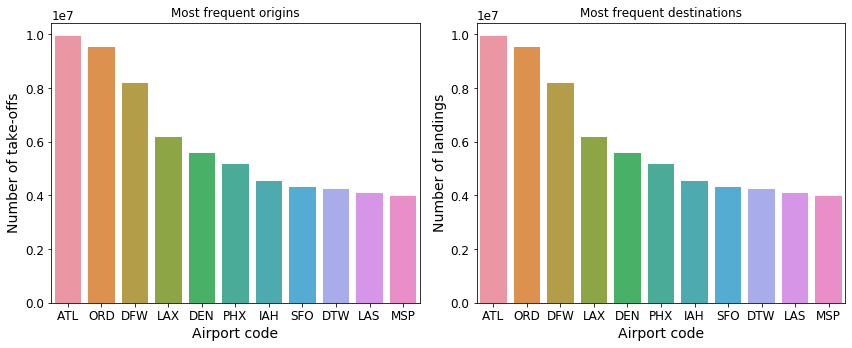##### When are flights scheduled to depart?¶

Let's look at the number of flights per day of week and month, as well as the number of flights per hour of day and day of week.

In :
df_filtered['Month'] = df_filtered.Month - 1  # to make it start from 0
df_filtered['DayOfWeek'] = df_filtered.DayOfWeek - 1  # to make it start from 0

# label them as categories
df_filtered.categorize(column='Month')
df_filtered.categorize(column='DayOfWeek')

# Helper lists for labelling the plots
label_month_list = ['Jan', 'Feb', 'Mar', 'Apr', 'May', 'Jun', 'Jul', 'Aug', 'Sep', 'Oct', 'Nov', 'Dec']
label_day_list = ['Mon', 'Tue', 'Wed', 'Thu', 'Fri', 'Sat', 'Sun']

In :
# Plot number of flights per month vs day of week
df_filtered.plot('Month', 'DayOfWeek', colorbar=True, colormap=cm_plusmin, figsize=(15, 5))
plt.xticks(np.arange(12), label_month_list)
plt.yticks(np.arange(7), label_day_list)
plt.xlabel('Month')
plt.ylabel('Day of week')
plt.tick_params(labelsize=12)
plt.show()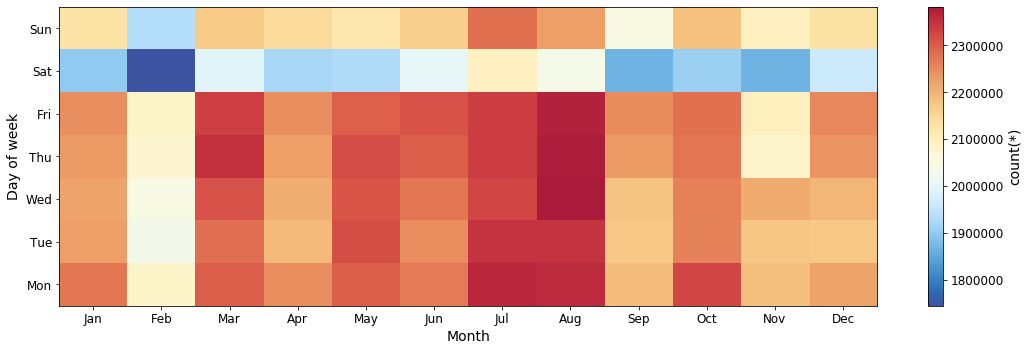In :
# Extract CRS Hour of departure
df_filtered['CRSDepHour'] = df_filtered.CRSDepTime // 100 % 24

# Treat as a categorical
df_filtered.categorize(column='CRSDepHour')

In :
# Plot number of flights per departure hour vs day of week
df_filtered.plot('CRSDepHour', 'DayOfWeek', colorbar=True, colormap=cm_plusmin, figsize=(15, 5))
plt.xticks(np.arange(24), np.arange(24))
plt.yticks(np.arange(7), label_day_list)
plt.tick_params(labelsize=12)
plt.show()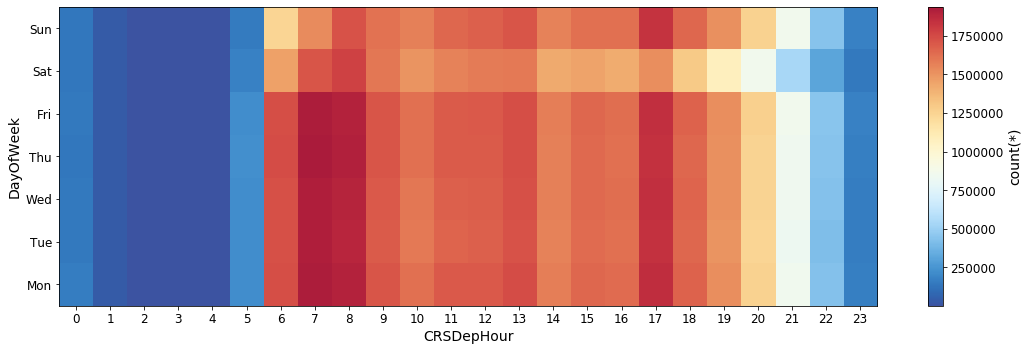##### Overall distributions of flight times, distances, and speed.¶

Now, let's look at the overall distribution of the flight durations, distances and speed of flight.

For some of the distributions the limits are adjusted to clip obvious outliers due to likely faulty data recordings.

In :
plt.figure(figsize=(18, 5))

plt.subplot(131)
df_filtered.plot1d('AirTime', limits=[0, 500], lw=3, shape=64)
plt.xlabel('AirTime [min]')

plt.subplot(132)
df_filtered.plot1d('Distance', limits='minmax', lw=3, shape=64)
plt.xlabel('Distance [miles]')

plt.subplot(133)
# Calculate the mean speed of the aircraft
df_filtered['Speed'] = df_filtered.Distance / (df_filtered.AirTime/60.)  # this is in miles per hour

df_filtered.plot1d('Speed', limits=[100, 700], lw=3, shape=64)
plt.xlabel('Speed [miles/hour]')

plt.tight_layout()
plt.show()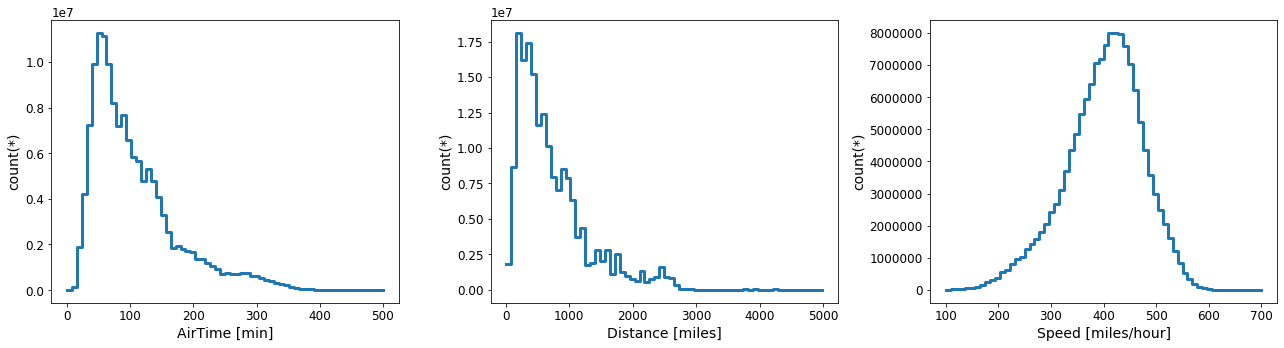##### Nearest neighbours¶

Out of curiositly, let's find which are the closest airports which had a regular connection between them.

In :
# Check if there are frequent flights at uber-short distances
df_filtered[df_filtered.Distance <= 20].Distance.value_counts(progress=True)

[########################################]:  100.00% elapsed time  :        0s =  0.0m =  0.0h

Out:
11    2710
17      46
18       5
16       3
12       2
10       2
19       1
8        1
6        1
dtype: int64

Ok rather large number of flights for distance of 11 miles. Let's find the airports in question.

In :
# Let's see the origins for this short route
df_filtered[df_filtered.Distance == 11.].Origin.value_counts()

Out:
SFO    1615
OAK    1042
JFK      36
LGA      17
dtype: int64

It looks like there are quite a large number of flights between SFO and OAK airports. Let's see how that connection evolved throughout the years.

In :
# How the number of the shortest destination flights evolved through the years
nf_sfo_oak = df_filtered[(df_filtered.Distance == 11.) & ((df_filtered.Origin=='SFO') | (df_filtered.Origin == 'OAK'))]['Year'].value_counts()
nf_jfk_lga = df_filtered[(df_filtered.Distance == 11.) & ((df_filtered.Origin=='JFK') | (df_filtered.Origin == 'LGA'))]['Year'].value_counts()

# plot it
fig, (ax1, ax2) = plt.subplots(nrows=1, ncols=2)
fig.set_size_inches(16, 4)

sns.barplot(nf_sfo_oak.index, nf_sfo_oak.values, ax=ax1)
sns.barplot(nf_jfk_lga.index, nf_jfk_lga.values, ax=ax2)

ax1.set_title('Number of flights between San Francisco and Oakland airpors')
ax2.set_title('Number of flights between John F. Kennedy and LaGuardia airpors')
ax1.set_ylabel('Number of flights')
ax1.set_xlabel('Year')
ax2.set_xlabel('Year')

plt.tight_layout()
plt.show()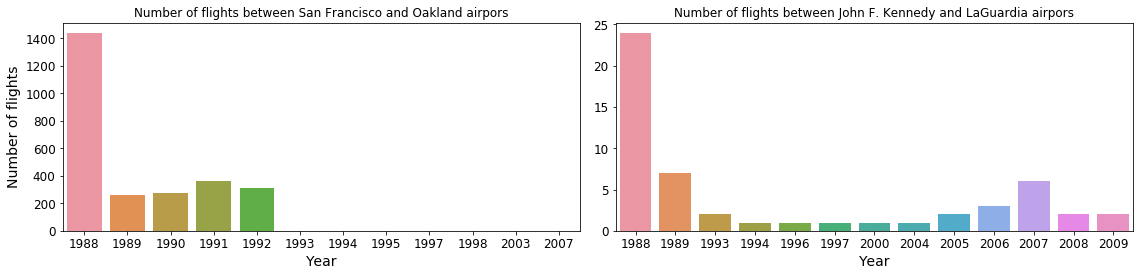In Oakland, it looks like a regular connection existed for 5 years! (at least).

In NYC, just a handful of flights scheduled over the years, probably private, or special services.

##### Long distance relationship¶

Let's find the most distant airports that have a regular connection.

In :
# Check if there are frequent flights at uber-long distances
df_filtered[df_filtered.Distance > 4900].Distance.value_counts(progress=True)

[########################################]:  100.00% elapsed time  :        0s =  0.0m =  0.0h

Out:
4962    13045
4983     4863
4963     2070
dtype: int64

So we have few common long distance relations. Let's examine them.

In :
# Group by Origin, Destination and Distance, and count the number of flights
df_filtered[df_filtered.Distance > 4900].groupby(by=['Origin', 'Dest', 'Distance'], agg={'Origin':'count'})

Out:
# Origin Dest Distance count
0EWR HNL 4962 6517
1EWR HNL 4963 1035
2HNL EWR 4962 6528
3HNL EWR 4963 1035
4HNL JFK 4983 2432
5JFK HNL 4983 2431

There are assentially two pairs of airports. Newark (EWR) --- Honolulu (HNL); and John F. Kennedy (JFK) --- Honolulu (HNL)

Notice that between EWR and HNL there are two sets of distances, which are very close to each other. We will examine this in turn.

Now let's look at the number of flights per year between these airport pairs.

In :
# Flight between Newark and Honolulu airport (the more common distance of 4962 miles)
nf_ewr_nhl = df_filtered[(df_filtered.Distance == 4962)]['Year'].value_counts()

plt.figure(figsize=(14, 4))
sns.barplot(nf_ewr_nhl.index, nf_ewr_nhl.values)
plt.title('Number of flights between Newark Liberty International and Honolulu International airpors')
plt.ylabel('Number of flights')
plt.xlabel('Year')
plt.show()

# Pretty much 2 per day since 1999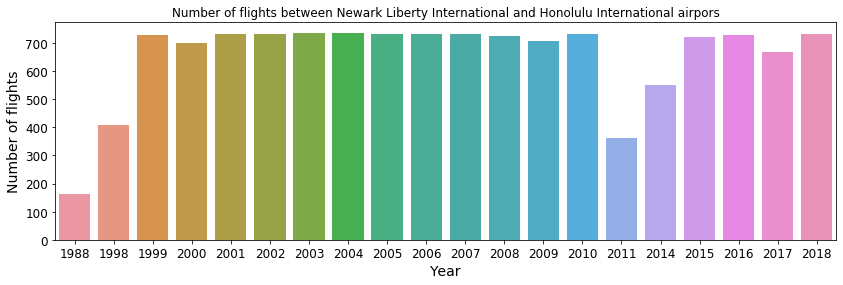In :
# Flight between Newark and Honolulu airport (the less common distance of 4963 miles)
nf_ewr_nhl = df_filtered[(df_filtered.Distance == 4963)]['Year'].value_counts()

plt.figure(figsize=(14, 4))
sns.barplot(nf_ewr_nhl.index, nf_ewr_nhl.values)
plt.title('Number of flights between Newark Liberty International and Honolulu International airpors')
plt.ylabel('Number of flights')
plt.xlabel('Year')
plt.show()

# Pretty much 2 per day since 1999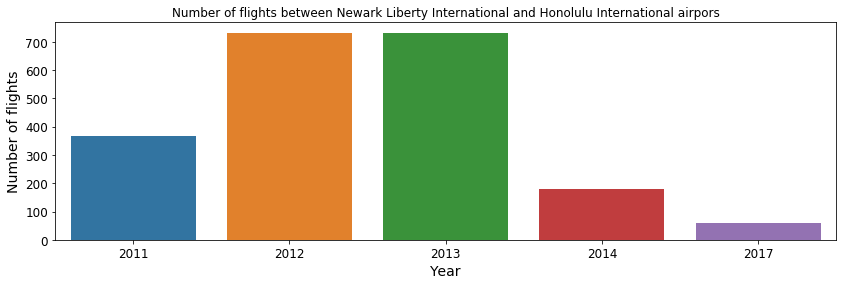The flights between Honolulu International Airport and Newark Liberty International should travel exactly 4962 miles. However, due to the tragedy in in 2001, the trajectory of the route has been changed slightly, accounting for the change in the distance flown. If we sum up the above two histograms, we can see that the service was never suspended: 2 flights per day since 1999!

In :
# Flight between Newark and Honolulu  airport (all distances)
nf_ewr_nhl = df_filtered[(df_filtered.Distance == 4962) |
(df_filtered.Distance == 4963)]['Year'].value_counts()

plt.figure(figsize=(14, 4))
sns.barplot(nf_ewr_nhl.index, nf_ewr_nhl.values)
plt.title('Number of flights between Newark Liberty International and Honolulu International airpors')
plt.ylabel('Number of flights')
plt.xlabel('Year')
plt.show()

# Pretty much 2 per day since 1999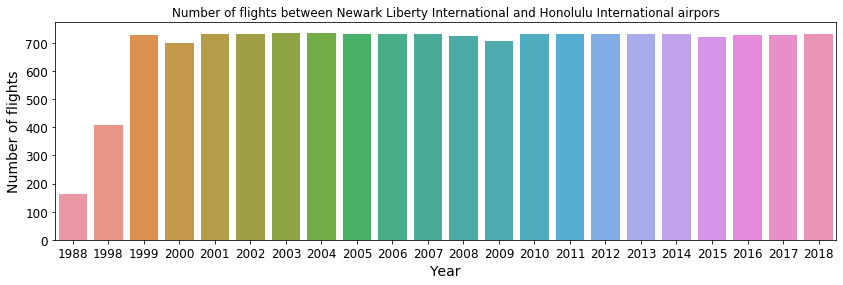Let's do the same, now of the right JFK --- HNL pair.

In :
# Flights between John F Kennedy and Honolulu Airpot
nf_jfk_nhl = df_filtered[(df_filtered.Distance == 4983)]['Year'].value_counts()

plt.figure(figsize=(14, 4))
sns.barplot(nf_jfk_nhl.index, nf_jfk_nhl.values)
plt.title('Number of flights between John F. Kennedy International and Honolulu International airpors')
plt.ylabel('Number of flights')
plt.xlabel('Year')
plt.show()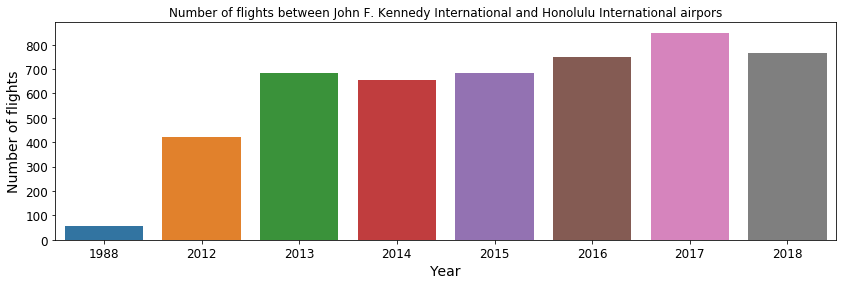Pretty much 2 flights per day since 2013 as well.

### Look at some aggregates of the data & identifying the central transport hubs¶

There are a lot of airports in this dataset. But most of them do no not contribute much to the over all traffic.

In :
# Start by checking the number of flights per origin
n_flights_per_origin = df_filtered.Origin.value_counts(progress=True)

# See how many of the airports "contribute" to the total traffic.
print('Total number of flights:', n_flights_per_origin.sum())
print('Total number of unique airports:', len(n_flights_per_origin))
_ = n_flights_per_origin[:10].sum() / n_flights_per_origin.sum() * 100
print('Percentage of total flights contributed by the 10 most frequent airports: %.1f%%' % (_))
_ = n_flights_per_origin[:30].sum() / n_flights_per_origin.sum() * 100
print('Percentage of total flights contributed by the 30 most frequent airports: %.1f%%' % (_))
_ = n_flights_per_origin[:50].sum() / n_flights_per_origin.sum() * 100
print('Percentage of total flights contributed by the 50 most frequent airports: %.1f%%' % (_))
_ = n_flights_per_origin[:100].sum() / n_flights_per_origin.sum() * 100
print('Percentage of total flights contributed by the 100 most frequent airports: %.1f%%' % (_))

[########################################]:  100.00% elapsed time  :        6s =  0.1m =  0.0h
Total number of flights: 183819525
Total number of unique airports: 411
Percentage of total flights contributed by the 10 most frequent airports: 33.6%
Percentage of total flights contributed by the 30 most frequent airports: 64.6%
Percentage of total flights contributed by the 50 most frequent airports: 80.1%
Percentage of total flights contributed by the 100 most frequent airports: 93.1%


Now we wanna turn our attention to just the dominant transport hubs, i.e. the more frequently used airports. To do this, let's construct a DataFrame in which we will aggregate some of the flight data that we are interested in, based on the point of Origin of each flight. We will also count the number of flights departing from each Origin.

From the this grouped DataFrame, let's filter out airports with less than 200_000 departures. The rationale is the following: for an airport to be considered frequently used, we require for it to have, on average, 20 destinations per day, one flight per day for each of the over 30 years. These criteria are of course arbitrary.

In :
# Group by origin, calculate some aggregate stats
df_group_by_origin = df_filtered.groupby(by='Origin', agg={'Year': 'count',
'Distance': ['mean', 'std'],
'TaxiIn': ['mean', 'std'],
'TaxiOut': ['mean', 'std'],
'DepDelay': ['mean', 'std'],
})

# Require to have at least 200k flights, to be considered a typical commercial airport
# (~20 destinations with one flight per day for at least 30 years)
df_group_by_origin = df_group_by_origin[(df_group_by_origin['count'] > 200_000)]
df_group_by_origin

Out:
# Origin count Distance_mean Distance_std TaxiIn_mean TaxiIn_std TaxiOut_mean TaxiOut_std DepDelay_mean DepDelay_std
0 EWR 3859089949.0571505347506683.2925150804604 6.838859406285781 5.251657487563603525.17696004873647616.31522793477969612.16040468644496 36.88187947317769
1 CVG 2236016609.4698910920137462.1946183756504 7.084379192896184 25.31351448946385216.22206424898427 14.0085288391816727.816483497436057 31.602297095284012
2 IAH 4536870823.7763056909279478.1311208961596 6.222613386537248 4.511241307472678 18.27043037853273 10.2270493731190878.016037724277812 29.73318498885663
3 CMH 1043900553.7959335185362419.276147623531247.491396621204861 7.516344340461818 12.4577564523662638.72102111843323 7.867450008695839 33.14990352504729
4 MSP 3991564781.2819729309113454.3176216823334 6.767775443591901 5.983795721994184 18.49446532073246310.7320726571499637.25238365298468 29.763247664698596
... ... ... ... ... ... ... ... ... ... ...
103ISP 217621 680.4168439626691399.459870518570145.340379240609876 15.1179934714557399.534550794316752 6.063978121339791 7.429246477090541 27.84409835266481
104LBB 236482 330.7310577549243111.3834323713817 5.91249803343452654.882674768768664 8.967333306178974 5.144326845523153 6.31462146038509 27.81948038801572
105MDW 2036806683.0181455671282456.4011863833172 5.485737155429885 5.139088077261766 12.0956779161914967.944049952532181 11.45466525003103630.028289049173726
106JAN 274483 402.2841451018825160.005796220183538.076626032355284 10.86861283952515410.9216058339844476.61651989414567957.065939519638525 31.954831690328525
107FAT 239846 419.3971923651009338.8946939867262 7.950092585296667 5.206262971708373 11.36442416995143 6.085021086488832 6.833798912193658 35.815952046865924

Using the aggregations, we can examine, for instance, which are the most far-reaching airports.

In :
# Sort the grouped dataframe by the mean distance, and show the top 10 rows.

Out:
# Origin count Distance_mean Distance_std TaxiIn_mean TaxiIn_std TaxiOut_mean TaxiOut_std DepDelay_mean DepDelay_std
0SJU 702358 1464.27 405.821 8.51326 6.78075 14.767 6.08924 8.07646 38.908
1HNL 1.01435e+06 1434.64 1461.09 6.24755 4.49869 14.1278 5.32919 3.01592 32.826
2JFK 2.37464e+06 1329.25 881.625 6.90835 9.6304 28.4205 17.3369 11.6521 39.1827
3SEA 3.10943e+06 1177.29 632 6.46298 4.91571 15.5762 6.61427 7.46982 28.2154
4ANC 569356 1171.8 796.034 5.14451 3.55129 12.8845 6.35645 5.93125 30.087
5OGG 389631 1118.23 1248.24 6.57531 4.92173 8.99997 4.78332 2.52952 34.3253
6SFO 4.31266e+06 1104.17 870.573 6.67325 5.17686 17.4708 7.7682 10.6094 32.9309
7LAX 6.17816e+06 1080.4 861.132 6.16223 4.77581 15.6503 7.52632 8.33092 28.1817
8MIA 2.18468e+06 998.621 502.731 7.6241 6.25082 18.2477 9.4347 10.0096 34.5715
9EWR 3.85909e+06 949.057 683.293 6.83886 5.25166 25.177 16.3152 12.1604 36.8819

The furthest reaching airports are of course those located in remote places like Hawaii, Alaska, or Puerto Rico. In addition to these, the largest central hubs like LA, NY, SF, Miami are also far-reaching.

Next, let's find the mean distance of all the mean distances in the grouped DataFrame. This gives a "natural" scale between big cities or populare destination for the U.S.; i.e. it gives a sense of the typical separation beteween important destinations.

In :
# Mean Distance
df_group_by_origin.Distance_mean.mean()
# Median distance
np.median(df_group_by_origin.Distance_mean.values)

Out:
597.9130442788901
##### Taxi times¶

Let's look at the distribution of mean taxi-in and taxi-out times for the frequent airports.

In :
# Taxing
plt.figure(figsize=(8, 4))
df_group_by_origin.plot1d('TaxiIn_mean', limits=[0, 30], lw=2, shape=64, label='Taxi-in')
df_group_by_origin.plot1d('TaxiOut_mean', limits=[0, 30], lw=2, shape=64, label='Taxi-out')
plt.xlabel('Taxi [min]')
plt.legend()
plt.show()

# Taxing out on average takes much longer than Taxing in:
# makes sense, you don't wait so much once the plane landf, but can often wait on "queue" before taking off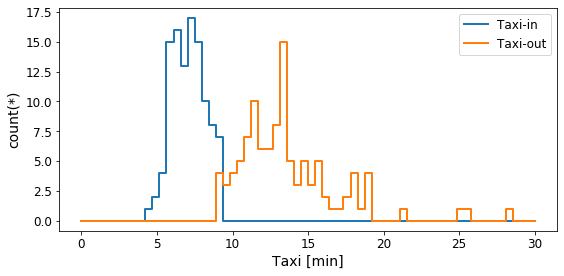Let's point fingers and find the airports with the shortest and longest taxi times.

In :
# Shortest taxi in
print('Shortest Taxi-In:')
display(
)

# lonest taxi in
print('Longest Taxi-In:')
display(
)

# shortest taxi out:
print('Shortest Taxi-out:')
display(
)

print('Longest Taxi-out:')
display(
)

# DAL - Dallas Love Field Airport (Texas)
# ICT - Wichita Dwight D. Eisenhower (Kansas)
# LBB - Lubbock Preston Smith International Airport (Texas)
# JFK - John F. Kennedy International Airport (NYC)

Shortest Taxi-In:

# Origin count Distance_mean Distance_std TaxiIn_mean TaxiIn_std TaxiOut_mean TaxiOut_std DepDelay_mean DepDelay_std
0DAL 1.49833e+06 380.81 270.675 4.30246 3.26236 9.5211 5.72323 9.53513 24.6417
Longest Taxi-In:

# Origin count Distance_mean Distance_std TaxiIn_mean TaxiIn_std TaxiOut_mean TaxiOut_std DepDelay_mean DepDelay_std
0ICT 309076 523.074 192.711 9.31726 9.83219 12.2629 8.00531 6.3479 34.5787
Shortest Taxi-out:

# Origin count Distance_mean Distance_std TaxiIn_mean TaxiIn_std TaxiOut_mean TaxiOut_std DepDelay_mean DepDelay_std
0LBB 236482 330.731 111.383 5.9125 4.88267 8.96733 5.14433 6.31462 27.8195
Longest Taxi-out:

# Origin count Distance_mean Distance_std TaxiIn_mean TaxiIn_std TaxiOut_mean TaxiOut_std DepDelay_mean DepDelay_std
0JFK 2.37464e+06 1329.25 881.625 6.90835 9.6304 28.4205 17.3369 11.6521 39.1827

## Exploratory Data Analysis of the frequent origins¶

To create a DataFrame containing only the most frequent origins, as selected above, do an inner join between the main DataFrame and the grouped DataFrame, from which the less frequently used airports are already filtered out.

In :
# Now only consider the flights emerging from the top 115 Origins.
df_top_origins = df_filtered.join(other=df_group_by_origin,
on='Origin',
how='inner',
rsuffix='_')


Now let's create a new virtual column (takes no memory!) to indicate whether a flight has been delayed or not. Note that such a column already exists regarding cancellations.

In :
# If a flight is being delayed
df_top_origins['Delayed'] = (df_top_origins.DepDelay > 0).astype('int')

##### Time evolution¶

Let's see how some of the flight properties change through time. To do this, let's group them by year.

In :
# group by year
df_group_by_year = df_top_origins.groupby(by='Year',
agg={'Year': 'count',
'Distance': ['mean', 'std'],
'TaxiIn': ['mean', 'std'],
'TaxiOut': ['mean', 'std'],
'DepDelay': ['mean', 'std', 'sum'],
'Cancelled': ['sum'],
'Delayed': ['sum'],
'Diverted': ['sum']})


How have the mean taxi times changed throughout the years. Note that these measurements were not recorded prior to 1995.

In :
plt.figure(figsize=(18, 4))

plt.subplot(121)
sns.barplot(df_group_by_year.Year.values, df_group_by_year.TaxiIn_mean.values)
plt.xticks(rotation='vertical')
plt.xlabel('Year')
plt.ylabel('Duration [min]')
plt.title('Mean Taxi-in duration')

plt.subplot(122)
sns.barplot(df_group_by_year.Year.values, df_group_by_year.TaxiOut_mean.values)
plt.xticks(rotation='vertical')
plt.xlabel('Year')
plt.title('Mean Taxi-out duration')

plt.tight_layout()
plt.show()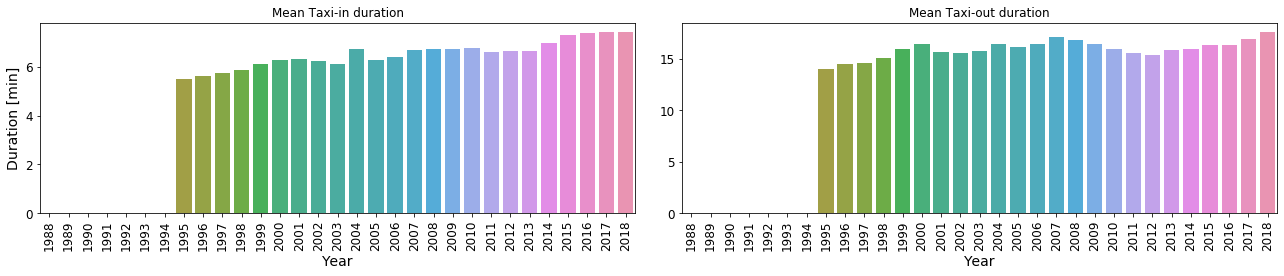The evolution of the mean distance over time. Gives an indication whether airports are connected with more distanant destinations over time.

In :
plt.figure(figsize=(12, 4))
sns.barplot(df_group_by_year.Year.values, df_group_by_year.Distance_mean.values)
plt.xticks(rotation='vertical')
plt.xlabel('Year')
plt.ylabel('Mean Distance [miles]')
plt.title('Mean distance traveled by the flights')
plt.show()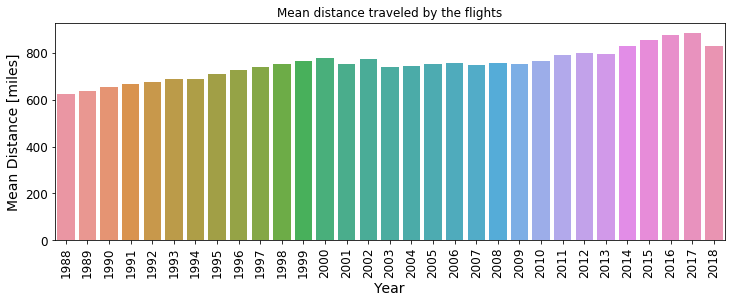Interesting.. the distance increases with time, then it becomes somewhat stable, and it increases again after 2014. Probably new destinations are being added or connected to each other.

### Cancellations and Delays¶

Now let's turn to something more interesting.. Let's look at the number of flight delays and calncellations over time.

In :
plt.figure(figsize=(14, 4))
sns.barplot(df_group_by_year.Year.values, df_group_by_year.Delayed_sum.values)
plt.xticks(rotation='vertical')
plt.xlabel('Year')
plt.ylabel('Delays')
plt.title('Number of delays per year')
plt.show()

plt.figure(figsize=(14, 4))
sns.barplot(df_group_by_year.Year.values, df_group_by_year.Cancelled_sum.values)
plt.xticks(rotation='vertical')
plt.xlabel('Year')
plt.ylabel('Cancellations')
plt.title('Number of cancellations per year')
plt.show()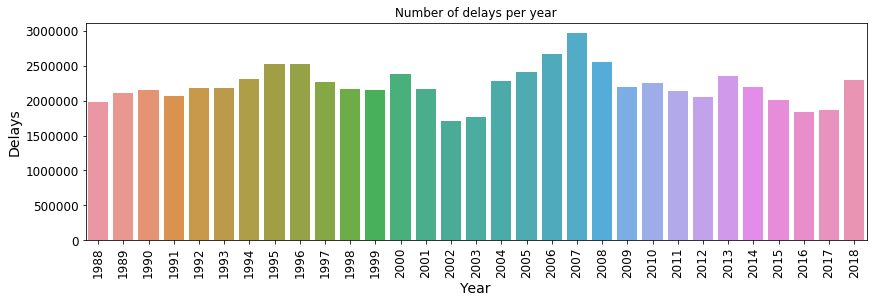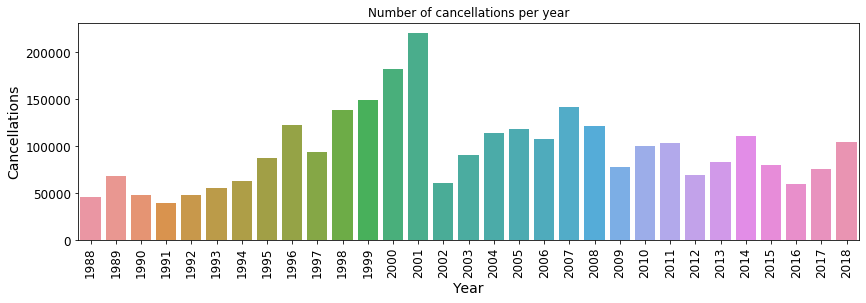Find the total number of cancellations per year for each cancellation type, for these most common airports.

First let's check if the number of cancellations and cancellation codes match:

In :
# Number of flights that have cancellation codes:
df_top_origins.CancellationCode.value_counts(progress=True)

[########################################]:  100.00% elapsed time  :        8s =  0.1m =  0.0h

Out:
missing    171269977
1091693
B             216133
A             147315
C              75065
D                257
dtype: int64

We see that there are two types of "missing" values in the case of Cancellation codes. One is an empty string, another is masked values. Let's look if either show any indication of cancelled flights.

In :
# There are cancellation codes that are empty strings. Let's see what they contain
df_top_origins[df_top_origins.CancellationCode==''].Cancelled.value_counts(progress=True)

# So in the current dataset, all cancellation codes that are empty strings are flights which were not cancelled.

[########################################]:  100.00% elapsed time  :        4s =  0.1m =  0.0h

Out:
0    1091693
dtype: int64

So in the current dataset, all cancellation codes that are empty strings are flights which were not cancelled.

Now let's do the same for the masked values.

In :
# Check for cancelled flights masked values of the cancellation codes
df_top_origins[df_top_origins.CancellationCode.ismissing()].Cancelled.value_counts(progress=True)

# So there are 2_547_952 flights which were cancelled, for which there is no cancellation code.

[########################################]:  100.00% elapsed time  :        3s =  0.1m =  0.0h

Out:
0    168722025
1      2547952
dtype: int64

So there are 2_547_952 flights which were cancelled, for which there is no cancellation code. We will interpret this as "reason unknown" and will simply ignore these.

Next up, let's check the total number of cancelled flights.

In :
# Check the total number of cancelled flights
df_top_origins.Cancelled.value_counts(progress=True)

[########################################]:  100.00% elapsed time  :        1s =  0.0m =  0.0h

Out:
0    169813731
1      2986709
dtype: int64

Let's check for consistency: the total number of cancelled flights should be equal to the sum of known cancellation codes (A, B, C, D) + the cancelled flights with unknown cancellation code.

In :
# Check for consistency
# Sum the value counts from the different cancellation codes + those of cancelled flights with missing codes
print(2547952 + 216133 + 147315 + 75065 + 257 - 2986709)

13


This means that there are 13 flights that do have a cancellation code, but where not marked as cancelled. Let's check this in another way: count the number of flights which were not cancelled, but have any sort of cancellation code.

In :
# This means that there are probably (13?) flights that do have a cancellation code which were not cancelled.
# let's verify that
((df_top_origins.Cancelled == 0) & ((df_top_origins.CancellationCode == 'A') |
(df_top_origins.CancellationCode == 'B') |
(df_top_origins.CancellationCode == 'C') |
(df_top_origins.CancellationCode == 'D'))).sum()
# This is such a small discrepancy, we decide to let is slide.
# In the following analysis of the cancellation code, we will ignore the unknown reason for the cancelled flights
# with unspecified cancellation code

Out:
array(13)

We recover the same number of flights (13) which do have a cancellation code, but were marked as not cancelled. This is such a small discrepancy, we decide to ignore it for the present analysis.

Now that we have all this figured out: Let's count the number of cancelled flights for each reason per year. Note that the cancellation code as a field in the dataset was introduced in 2003, so we don't need to consider earlier years for this gruoping.

In :
# Now groupby year and a cancellation code
# The cancellation code was introduced in the database after 2002, hence the filtering
df_year_cancel = df_top_origins[df_top_origins.Year > 2002].groupby(by=['Year', 'CancellationCode'],
agg={'Cancelled': ['sum']})

# Keep only the portion of the DataFrame that contains a proper cancellation code:
df_year_cancel = df_year_cancel[~((df_year_cancel.CancellationCode == '') |
(df_year_cancel.CancellationCode == 'null') )]


Let's add more meaningful names to the cancellation codes. The designations come from the documentation available at the archive from which this dataset was obtained.

In :
# Add more meaningful names to the cancellation codes
canc_code_mapper = {'A': 'carrier',
'B': 'weather',
'C': 'National Airspace System',
'D': 'Security'}
df_year_cancel['CancellationCode_'] = df_year_cancel.CancellationCode.map(canc_code_mapper, allow_missing=False)


Now let's make a plot showing the number of cancellations, per season, per year. The seaborn library is quite useful for this.

In :
plt.figure(figsize=(18, 5))
sns.barplot(x='Year',
y='Cancelled_sum',
hue='CancellationCode_',
data=df_year_cancel.to_pandas_df(virtual=True),
palette=sns.color_palette(['C0', 'C1', 'C2', 'C3', 'C6']))

plt.yscale('log')
plt.xticks(rotation='vertical')
plt.ylabel('Number of cancellations')
plt.show()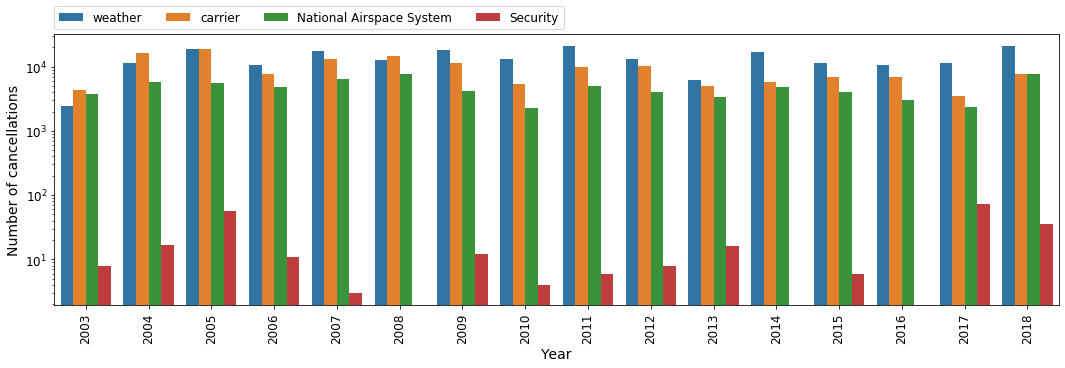Let's point fingers again and see at which airports flights experience the most delays and cancellations.

In :
# groupby origin to investigate popular cancellations
df_group_by_origin_cancel = df_top_origins.groupby(by='Origin').agg({'Delayed': ['count', 'sum'],
'Cancelled': ['sum']})


Let's add a couple of new features: the percentage of flights that are delayed or cancelled, for each Origin. Then plot the results.

In :
df_group_by_origin_cancel['delayed_perc'] = df_group_by_origin_cancel.Delayed_sum/df_group_by_origin_cancel['count'] * 100.
df_group_by_origin_cancel['cancelled_perc'] = df_group_by_origin_cancel.Cancelled_sum/df_group_by_origin_cancel['count'] * 100.

plt.figure(figsize=(14, 4))

plt.subplot(121)
sns.barplot(df_group_by_origin_cancel.sort(by='delayed_perc', ascending=False)['Origin'].tolist()[:11],
df_group_by_origin_cancel.sort(by='delayed_perc', ascending=False)['delayed_perc'].tolist()[:11])
# plt.xticks(rotation='vertical')
plt.xlabel('Origin airport code')
plt.ylabel('Delayed flights (%)')
plt.title('Origin of most commonly delayed flights')

plt.subplot(122)
sns.barplot(df_group_by_origin_cancel.sort(by='cancelled_perc', ascending=False)['Origin'].tolist()[:11],
df_group_by_origin_cancel.sort(by='cancelled_perc', ascending=False)['cancelled_perc'].tolist()[:11])
# plt.xticks(rotation='vertical')
plt.xlabel('Origin airport code')
plt.ylabel('Cancelled flights (%)')
plt.title('Origin of most commonly cancelled flights')

plt.tight_layout()
plt.show()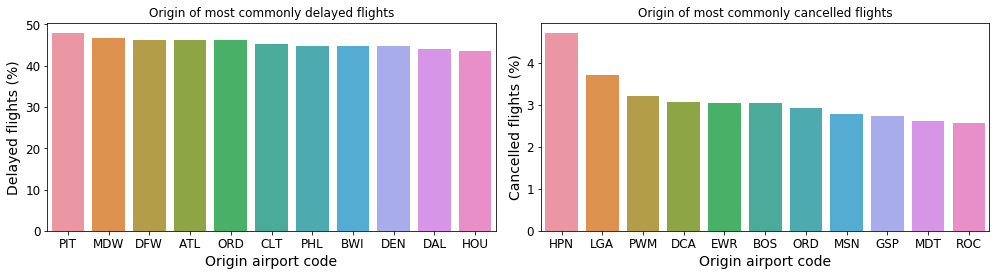Pittsburgh International (PIT), Chicago Midway International (MDW) and Dallas International (DFW) are the top 3 airports with the largest percentage of delayed flights.

Westchester County Airport (HPN), LaGuardia Airport (LGA) and Portland International Jetport (PWM) are the top 3 airports with the largest percentage of cancelled flights.

Now let's reaaaaly point figures and figure out which Carriers experience the most delays and cancellations, regardless of the point of origin.

In :
# groupby origin to investigate popular cancellations
df_group_by_carrier_cancel = df_top_origins.groupby(by='UniqueCarrier', agg={'Delayed': ['count', 'sum'],
'Cancelled': ['sum']})

# Add new features: percentage of flights cancelled or delayed
df_group_by_carrier_cancel['delayed_perc'] = df_group_by_carrier_cancel.Delayed_sum/df_group_by_carrier_cancel['count'] * 100.
df_group_by_carrier_cancel['cancelled_perc'] = df_group_by_carrier_cancel.Cancelled_sum/df_group_by_carrier_cancel['count'] * 100.


To attach the carrier names we will use tha airline carrier auxiliary table from http://stat-computing.org/dataexpo/2009/supplemental-data.html. Now, this website is rather old, so it is possible tha some airlines have merged or for some reason has obtained new code, or maybe new airlines have emerged. Bottom line is, it may not be complete, but let's try it out here anyway.

In :
# Load the carrier name data
aux_df_carrers = vaex.from_csv('./data/aux-carriers.csv', copy_index=False)

# Create a mapper
mapper_carriers = dict(zip(aux_df_carrers.Code.values, aux_df_carrers.Description.values))

# Do the mapping
df_group_by_carrier_cancel['CarrierName'] = df_group_by_carrier_cancel.UniqueCarrier.map(mapper=mapper_carriers)

In :
# Some of the carriers have very long names, so let's shorten them a bit
df_group_by_carrier_cancel['CarrierName_short'] = df_group_by_carrier_cancel.CarrierName.str.slice(start=0, stop=23)

In :
plt.figure(figsize=(14, 6))

plt.subplot(121)
sns.barplot(df_group_by_carrier_cancel.sort(by='delayed_perc', ascending=False)['delayed_perc'].tolist()[:11],
df_group_by_carrier_cancel.sort(by='delayed_perc', ascending=False)['CarrierName_short'].tolist()[:11])
plt.ylabel('Carrier')
plt.xlabel('% of delayed flights')
plt.title('Airlines with the most delays')

plt.subplot(122)
sns.barplot(df_group_by_carrier_cancel.sort(by='cancelled_perc', ascending=False)['cancelled_perc'].tolist()[:11],
df_group_by_carrier_cancel.sort(by='cancelled_perc', ascending=False)['CarrierName_short'].tolist()[:11])
plt.ylabel('Carrier')
plt.xlabel('% of cancelled flights')
plt.title('Airlines with the most cancellations')

plt.tight_layout()
plt.show()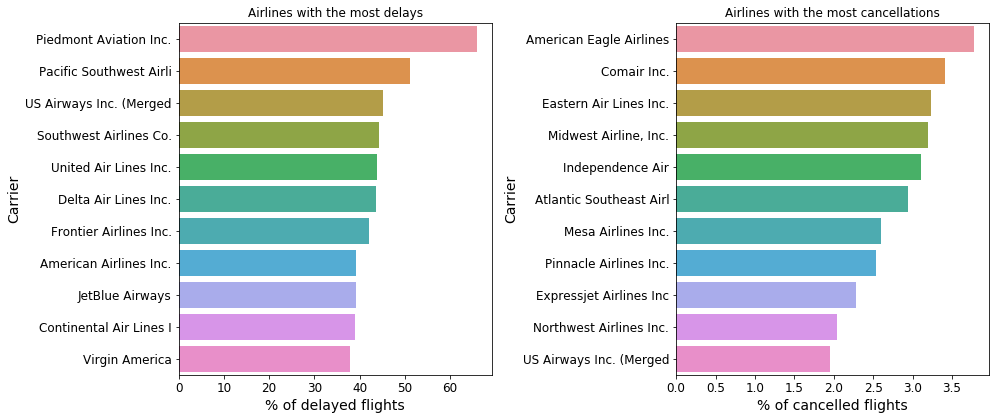Enough of pointing fingers, let's give some kudos. Let's determine which airlines have the fewest delays and cancellations.

In :
plt.figure(figsize=(14, 6))

plt.subplot(121)
sns.barplot(df_group_by_carrier_cancel.sort(by='delayed_perc', ascending=True)['delayed_perc'].tolist()[:11],
df_group_by_carrier_cancel.sort(by='delayed_perc', ascending=True)['CarrierName_short'].tolist()[:11])
plt.ylabel('Carrier')
plt.xlabel('% of delayed flights')
plt.title('Airlines with the fewest delays')

plt.subplot(122)
sns.barplot(df_group_by_carrier_cancel.sort(by='cancelled_perc', ascending=True)['cancelled_perc'].tolist()[:11],
df_group_by_carrier_cancel.sort(by='cancelled_perc', ascending=True)['CarrierName_short'].tolist()[:11])
plt.ylabel('Carrier')
plt.xlabel('% of cancelled flights')
plt.title('Airlines with the fewest cancellations')

plt.tight_layout()
plt.show()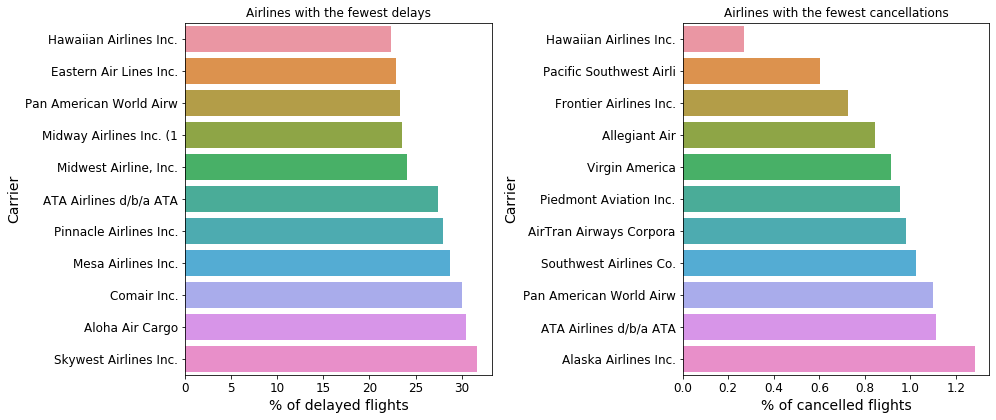"Safest" airlies in terms of delays or cancellations are the Hawaian. Makes sense, not much traffic jam happening on the islands, and if flights go outside, they travel a long way to they are probably better cared for.

##### More connected than ever¶

Next up, let's see the mean number of unique destinations each airport connects to, and how this number evoluves through time.

In :
# Aggregate the number of unique destination for each origin for each year
tmp_group = df_top_origins.groupby(by=['Year', 'Origin'], agg={'Year': 'count',
'Dest': [vaex.agg.nunique(df_top_origins.Dest,
dropna=True)]})
# Calculate the mean number of destinations for all origins per year
df_mean_num_dest = tmp_group.groupby('Year').agg({'Dest_nunique': ['mean']})

In :
plt.figure(figsize=(14, 4))
sns.barplot(x=df_mean_num_dest.Year.values, y=df_mean_num_dest.Dest_nunique_mean.values)
plt.xticks(rotation='vertical')
plt.xlabel('Year')
plt.ylabel('Mean number of destinations')
plt.show()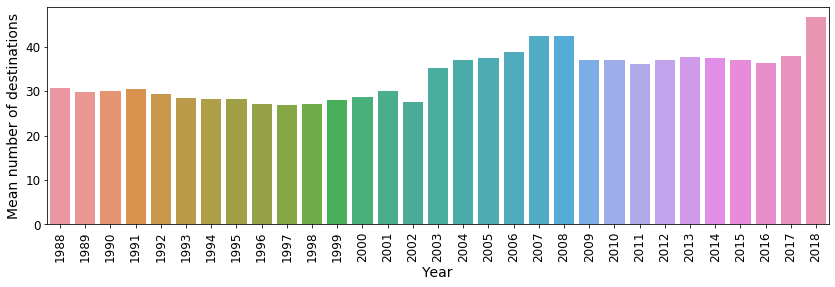A surge of new destinations in 2018! The world is getting more connected than ever.

##### Airplane performance¶

Let's take the auxiliary plane data from http://stat-computing.org/dataexpo/2009/supplemental-data.html and look at the typical performance of the aircraf used.

Warning: this data is certainly out of date by now.

In :
# Load the data about the airplane models
aux_df_planes = vaex.from_csv(filename_or_buffer='./data/aux-plane-data.csv', copy_index=False)

# A more convenient display of the model/type of each plane
aux_df_planes['model_type'] = aux_df_planes.manufacturer + ' ' + aux_df_planes.model

In :
# Join to the auxiliary plance data to the _df_top_origins DataFrame
df_top_origins_aux = df_top_origins.join(other=aux_df_planes,
left_on='TailNum',
right_on='tailnum',
how='left',
rsuffix='_')


Let's make a group based on the model type of the aircrafs used. We are interested in the typical speed and distance travelled by each model.

In :
# Group by model type
df_model_type = df_top_origins_aux.groupby(by='model_type',
agg={'Speed': 'mean', 'Distance': 'mean'})


Let's display the fastest and furthest reaching aircraft models (on average)

In :
# Sort and plot the top 11 fastest and far reaching aircraft.
model_top_speed = df_model_type.sort(by='Speed', ascending=False)[['model_type', 'Speed']]
model_top_distance = df_model_type.sort(by='Distance', ascending=False)[['model_type', 'Distance']]

plt.figure(figsize=(16, 5))

plt.subplot(121)
sns.barplot(x=model_top_distance.Distance[:11].tolist(),
y=model_top_distance.model_type[:11].tolist())
plt.xlabel('Mean distance [miles]')

plt.subplot(122)
sns.barplot(x=model_top_speed.Speed[:11].tolist(),
y=model_top_speed.model_type[:11].tolist())
plt.xlabel('Mean speed [miles/hour]')

plt.tight_layout()
plt.show()